# Relativistic Lagrangian for a charged particle in electromagnetic field.

The Lagrangian describes the dynamics of the system and is used in the Lagrangian formulation of classical mechanics to derive the equations of motion of the particle. The principle of least action is applied to the Lagrangian to derive the Euler-Lagrange equations, which are used to solve for the path of the particle in space and time.

1. Free particle

The equation of motion is

Consider x component ,

dpx/dt = 0

or, dmvx/dt =0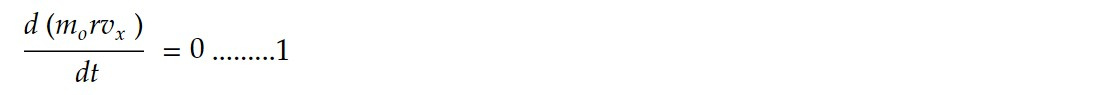Let us note that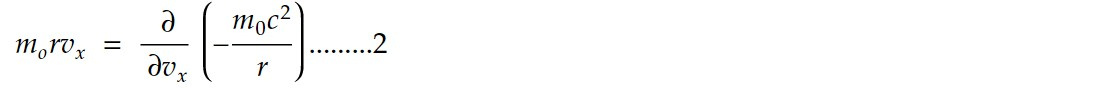So equation 1 becomes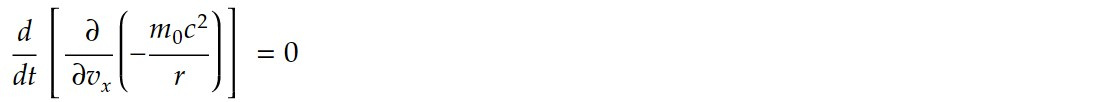This is similar to Lagrangian equation for free particle. So,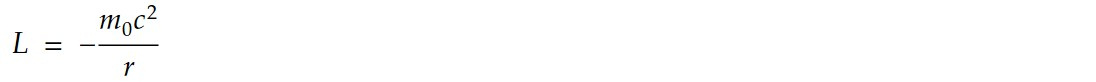2. Particle interacting with electromagnetic field.

In the Lagrangian formulation of mechanics, the motion of a particle interacting with an electromagnetic field is described by the Lagrangian of the system. The Lagrangian is a function of the particle's position, velocity, and time, as well as the electromagnetic field. The equation of motion is given byTaking x component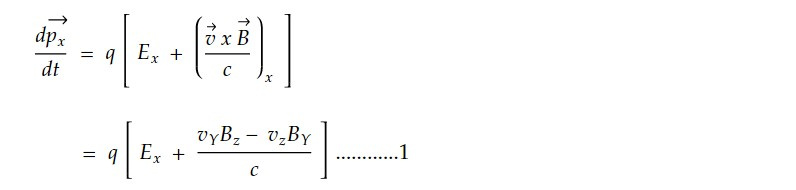Let us express Ex and Bx in terms of scalar and vector potential, then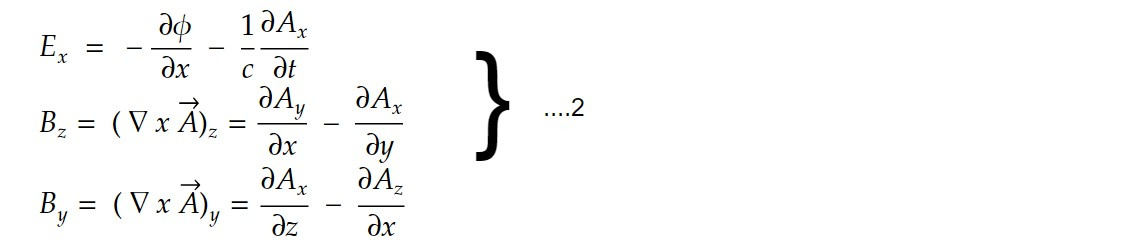Substituting 2 in 1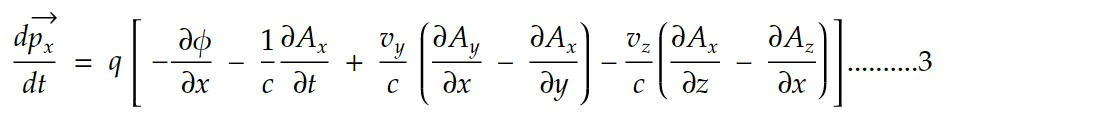Here the vector potential is given bySubstituting eq 4 in 3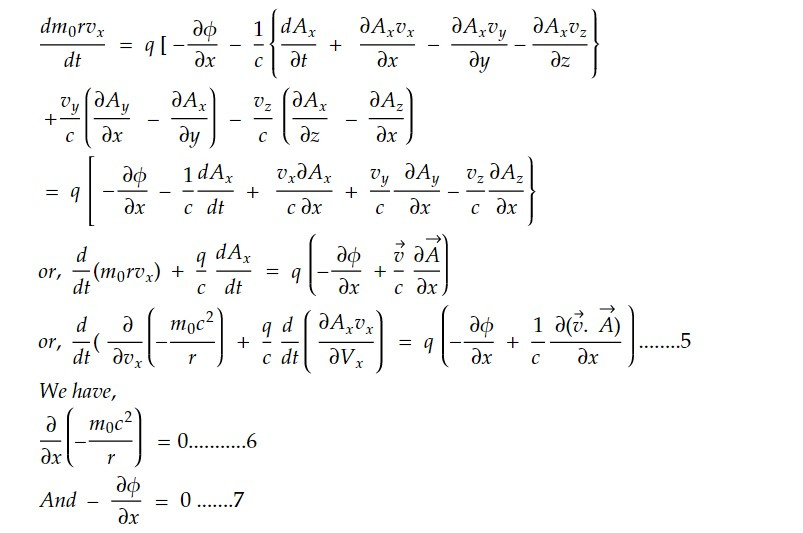Adding eq 6 on the right side of eq 5 and eq 7 to the left side of eq 5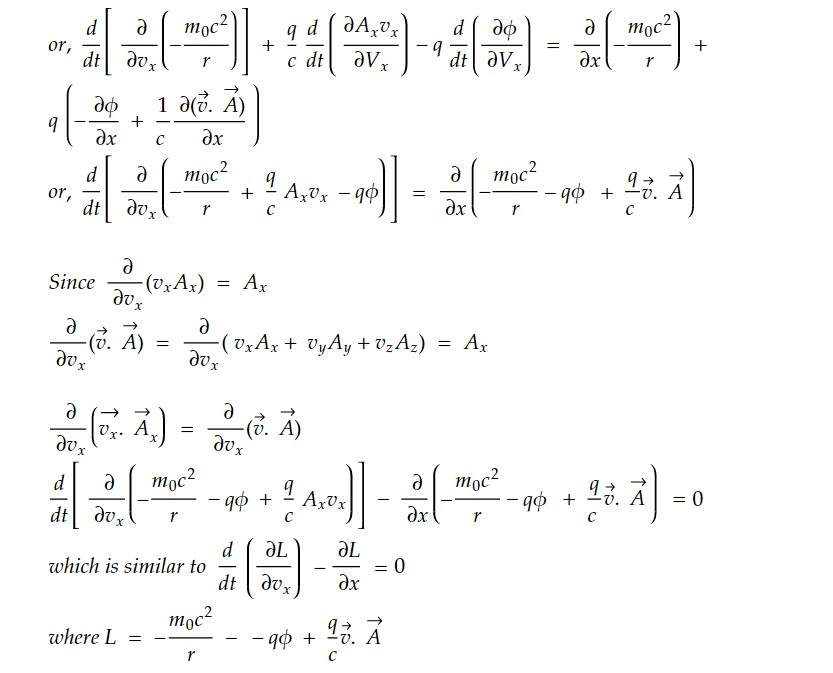The Lagrangian formulation is a powerful tool for analyzing the behavior of particles in electromagnetic fields, and it is used extensively in the study of particle physics, condensed matter physics, and other areas of physics and engineering.

This note is a part of the Physics Repository.